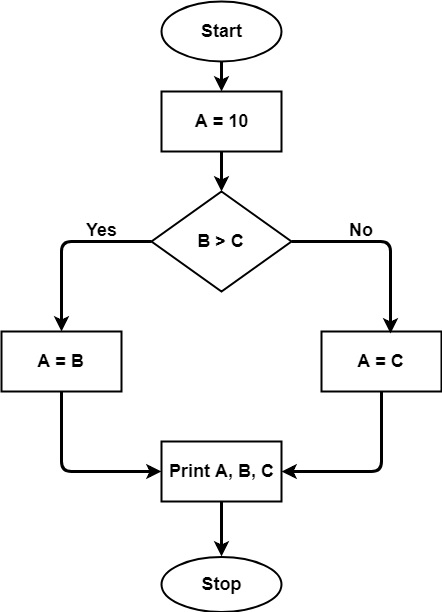# Mccabe's Cyclomatic Complexity: Calculate with Flow Graph (Example)

Software TestingAutomation TestingTesting Tools

## What is a Software Metric, exactly?

A quantitative indication of the size, dimension, or capacity of an attribute of a product or process is called as measurement. A software metric is a numerical measure of an attribute that a software system has in terms of Cost, Quality, Size, and Schedule.

Example

• Measure - Number of Errors
• Metrics - Number of Errors found per person

## Cyclomatic Complexity in Testing of Software

The number of linearly independent pathways in a code segment is measured in terms of cyclomatic complexity. It's a software statistic that shows how complicated a program is. It is calculated using the program's Control Flow Graph.

The nodes in the flow graph are the smallest sets of commands in a program, and a directed edge connects the two nodes, specify whether the second command should be executed immediately after the first. In 1976, Thomas J. McCabe created this statistic, which is based on a control flow model of the program. A program's control flow is represented as a graph with nodes and edges.

Nodes represent processing activities in the graph, whereas edges reflect control flow between nodes.

For example, if there are no control flow statements in the source code, the cyclomatic complexity is 1 and the source code only has one path. Similarly, if there is only one if condition in the source code, the cyclomatic complexity will be two because there will be two pathways, one for yes and the other for false. The directed graph inside the control flow is the edge connecting two basic blocks of the program mathematically, as control may transfer from first to second.

As a result, cyclomatic complexity M is defined as,

M = E – N + 2P

where E equals the number of control flow graph edges

The number of nodes in the control flow graph is denoted by N.

P stands for the number of interconnected components.

The following steps should be followed when calculating cyclomatic complexity and designing test cases −

• From code, create a graph containing nodes and edges.
• Independent pathways are identified.
• Calculation of Cyclomatic Complexity
• Creating Test Cases

As an example, consider the following code section −

A = 10
IF B > C THEN
A = B
ELSE
A = C
ENDIF
Print A
Print B
Print C

## Properties Cyclomatic Complexity

Cyclomatic complexity has the following properties −

Properties of cyclomatic complexity are as follows −

• V (G) >=1 is the graph's maximum number of independent pathways.
• V (G) >=1
• If V (G) = 1, G will have only one path.
• Reduce the complexity to ten.

Graph illustrating the above-mentioned code's control flow −The control flow graph will be used to calculate the cyclomatic complexity for the above code. Because there are seven shapes (nodes) and seven lines (edges) in the graph, the cyclomatic complexity is 7-7+2 = 2.

## More on V (G)

If the program is short, cyclomatic complexity can be estimated manually. If the software is particularly sophisticated, automated tools must be utilized because many flow graphs are involved. The team can come to a conclusion about the actions that need to be made for measurement based on the complexity number.

The table below summarizes the complexity number and its associated meaning for v (G) −

Complexity NumberMeaning
1-10
• Structured and well written code
• High Testability
• Cost and Effort is less
10-20
• Complex Code
• Medium Testability
• Cost and effort is Medium
20-40
• Very complex Code
• Low Testability
• Cost and Effort are high
>40
• Not at all testable
• Very high Cost and Effort

## Application of Cyclomatic Complexity

• Developers and testers have found that determining the independent path executions is really useful.

• It can verify that each path has been tried at least once. As a result, it will be easier to concentrate on paths that have yet to be discovered.

• It is possible to improve code coverage.

• It is possible to assess the program's risk.

The adoption of these indicators earlier in the program aids in the reduction of risks.

## Cyclomatic Complexity Benefits

• It can be used as a quality indicator, indicating the relative complexity of different designs.

• It can compute at a faster rate than Halstead's metrics.

• It is used to determine the least amount of effort required and the optimal areas of concentrate for testing.

• It has the ability to direct the testing procedure.

• It is simple to use.

## Cyclomatic Complexity Drawbacks

• It is a measure of the control complexity of the program, not the data complexity.

• Nested conditional structures are more difficult to comprehend than non-nested conditional structures in this regard.

• It may offer a deceptive figure in the case of basic comparisons and decision structures.

## Cyclomatic Complexity Calculation Methods

There are numerous tools available for determining the application's complexity. For specific technology, some complexity calculation methods are employed. The number of decision points of a program can be used to determine its complexity. If, for, for-each, while, do, catch, and case statements in source code are the decision points.

These are some examples of tools.

• OCLient - C and Related Languages Static Code Analyzer

• Reflector Add-In - Metrics for.NET assemblies

• Find metrics in Java-related apps with GMetrics.

## Conclusion

Cyclomatic Complexity is a software statistic that may be used in both structured and unstructured testing. It is mostly used to assess a program's complexity. The complexity of the software increases as the number of choice points increases. If a program's complexity is large, the likelihood of error is considerable, as is the time required for maintenance and troubleshooting.